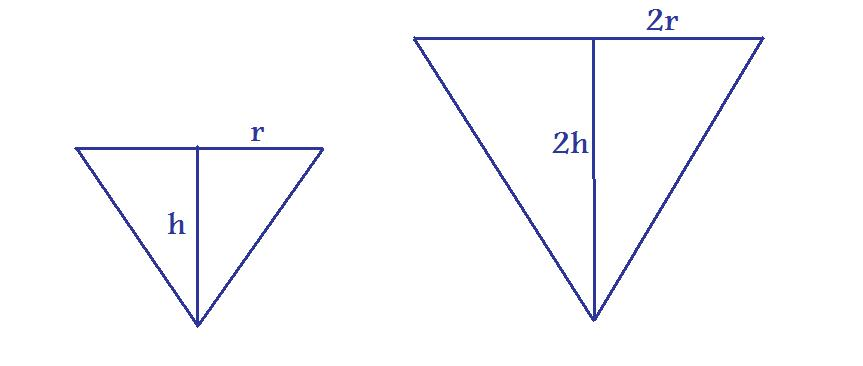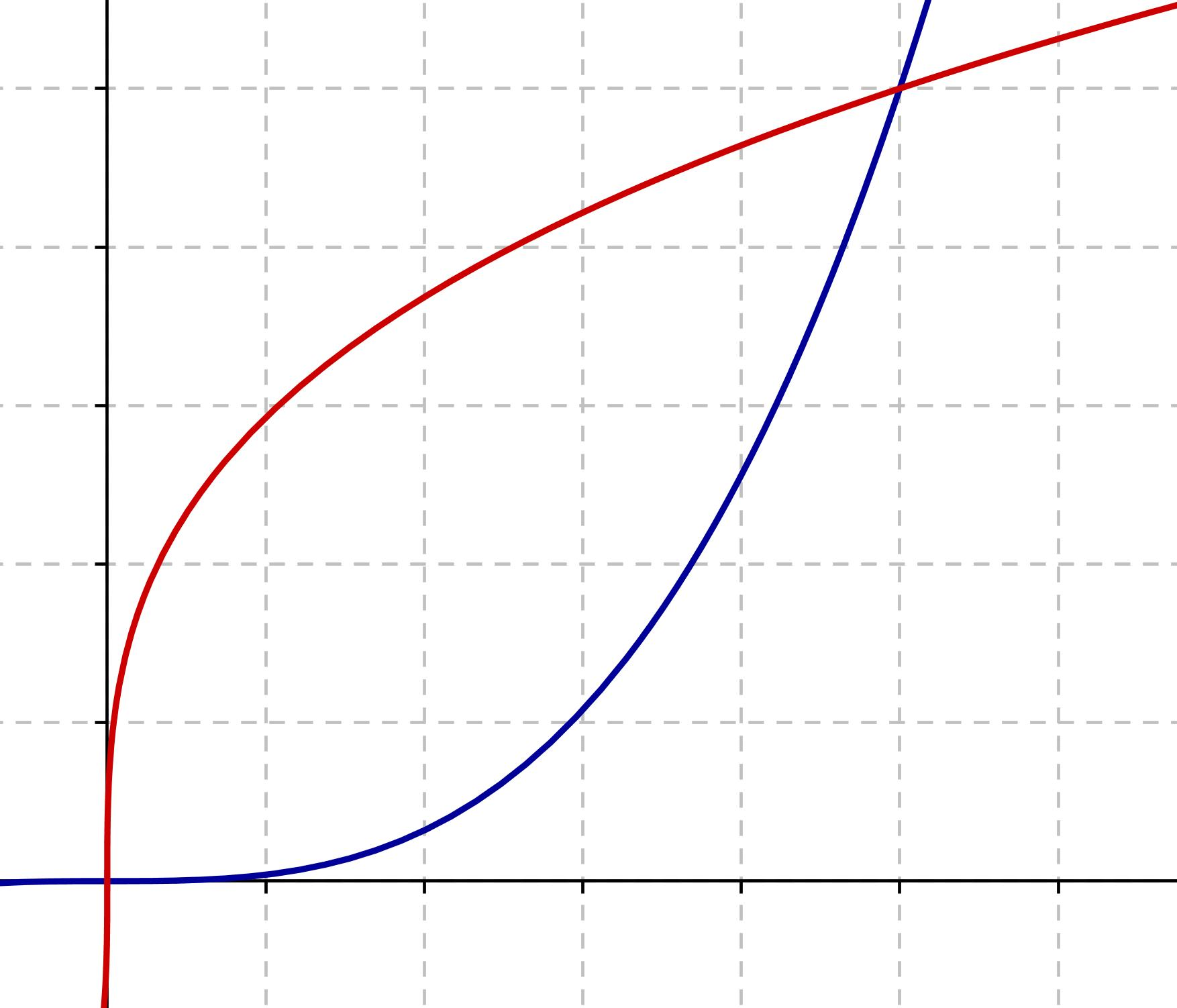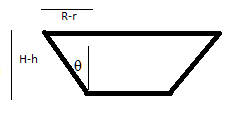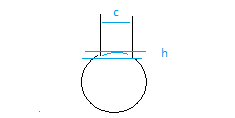#### You may also like### Ball Packing

If a ball is rolled into the corner of a room how far is its centre from the corner?### Three Balls

A circle has centre O and angle POR = angle QOR. Construct tangents at P and Q meeting at T. Draw a circle with diameter OT. Do P and Q lie inside, or on, or outside this circle?### In a Spin

What is the volume of the solid formed by rotating this right angled triangle about the hypotenuse?

# Fill Me up Too

##### Age 14 to 16Challenge Level

Well done to those of you who submitted solutions - this question is harder than it looks!

Tom from the Norwich School, gave some good explanations of the first section:

We know that the volume of a cone is given by $V_1=\frac {\pi}{3} r^2 h$.

1) We need to calculate the relationship between the height of the cone and the radius of its base.

Suppose we increase the height of our cone, and keep the proportions, i.e. the ratio of height to the radius, the same. Let $y$ be the height of our new cone, and $x$ be  the radius. The gradient, $\frac{h}{r}$, is therefore constant, so the relationship between $y$ and $x$ is given by:
\begin{align*} y &= \frac{h}{r}x \\ \text{If we set } y=2h &\Rightarrow 2h=\frac{h}{r}x \\\Rightarrow x& =2r \end{align*}From this, we are able to find the new volume:$$V_2 = 2^3 \bigg(\frac {\pi}{3} r^2 h \bigg)=2^3 V_1$$

2) If the height had trebled, then we use the same method:

\begin{align*} y &= \frac{h}{r}x \\ \text{If we set } y=3h &\Rightarrow 3h=\frac{h}{r}x \\\Rightarrow x& =3r \end{align*} and so $$V_2 = 3^3V_1$$

3) Noticing a pattern, we see that if the height had increased by a factor of n, then $$V_n = n^3 \bigg(\frac {\pi}{3} r^2 h \bigg)=n^3 V_1$$

4) Suppose we want to double the volume by changing the height (not forgetting the radius changes too!)i.e. $$V_2 = \frac {\pi}{3} r_2^2 h_2 = 2V_1 = \frac {\pi}{3} r_1^2 h_1$$

If $h_2 = kh_1$, then we know the radius also increases like $r_2 = kr_1$. Therefore \begin{align*}V_2 = k^3V_1 \Rightarrow k &= \sqrt{2} \\\text{ i.e. } h_2 &= \sqrt{2}h_1 \end{align*} Similarly, if we want to treble the volume, we should increase the height by a factor of $\sqrt{3}$. In general, if we want to increase the volume by a factor of n, we should increase the height by a factor of $\sqrt{n}$.

Cecil sent us graphs that demonstrated the cubic relationship:
5) The blue line is a graph of volume against height, and the red line is a graph of height against volume. We can see they're reflections of each other in the line $v=h$.Extension Challenge: Martin from All Saints School wrote to tell us this explanation about the graph for a pint glass.

The graph of a pint glass will have a steeper gradient at the start than that of the cone as the volume will rise more quickly and the height more slowly because there is a larger surface area at the bottom. However, the total volume and total height will be smaller than the cone.

Robert from Bishop Tonnos High School, Canada calculated the volume of a conical flask.

If you remove a cone of height h from the top of a larger cone (with the same proportions) of height H, the volume of the remaining object, a frustum, is $V = \frac{1}{3}\pi\tan^2{\theta}(H^3 - h^3)$ where $\theta$ is the angle between the vertical and the side. (Trigonometry implies $\tan(\theta) = \frac{H}{R}$, where $R$ is the radius of the large cone.) This is tricky to calculate, as we can't measure $H$ or $h$ easily. Robert noticed that $$\tan(\theta) = \frac{R-r}{H-h}$$ where r in the radius of the small cone.Denote the height of the frustam $H_f$ and the height of the cylindrical section $H_c$. As we're now considering part of a cone standing on its base, let $h$ be the level of the water. (NB $h$ is equivalent to $H-h$ in the previous formulae as it's upside down!)  the  The formula for the amount of water is the vessel is:

$$V = \left\{\begin{array}{l l}\frac{1}{3} \pi\frac{(R-r)^2}{H_f^2}(H_f^3 - (H_f-h)^3) & \quad h\leq H_f\\\frac{1}{3} \pi\frac{(R-r)^2}{H_f} + \pi r^2(h-H_f) & \quad h > H_f \\ \end{array} \right.$$

where the first term in the second equation is the frustum term and the second is the cylinder term.

Spherical vessel:

To derive the volume of a spherical vessel requires integration, which you'll learn at A-level, so don't worry if you don't understand this, it's getting really complicated!

The volume of a spherical cap cut from a sphere of radius $a$ is given by:

$$V= \frac{1}{6}\pi h(3b^2+h^2)$$ where $h$ is its height and $b^2 = 2ah - h^2$. We can use this result to find the volume of a spherical vessel. Suppose our vessel is a sphere with a spherical cap of height h removed, and a cylinder of radius c attached.We can therefore write an equation for the volume of the vessel. It will again be different depending on whether the height of the water, $w$ is greater or less than $2a - h$ (the point where the water will start filling the cylinder instead of the sphere). For $w < 2a-h$, the volume is itself a spherical cap of height w.

$$V_{vessel} =\left\{\begin{array}{l l} \frac{1}{6}\pi h(3b^2+w^2) & \quad w\leq 2a - h \\ S + \pi c^2(w-(2a-h) & \quad w > 2a-h \\ \end{array} \right.$$

where we've written the volume of the truncated sphere as $S$, where $$S = \frac{4\pi}{3}a^3 - \frac{1}{6}\pi h(3b^2+h^2)$$